JEE  >  Test: Linear Differential Equations

# Test: Linear Differential Equations

Test Description

## 10 Questions MCQ Test Mathematics (Maths) Class 12 | Test: Linear Differential Equations

Test: Linear Differential Equations for JEE 2022 is part of Mathematics (Maths) Class 12 preparation. The Test: Linear Differential Equations questions and answers have been prepared according to the JEE exam syllabus.The Test: Linear Differential Equations MCQs are made for JEE 2022 Exam. Find important definitions, questions, notes, meanings, examples, exercises, MCQs and online tests for Test: Linear Differential Equations below.
Solutions of Test: Linear Differential Equations questions in English are available as part of our Mathematics (Maths) Class 12 for JEE & Test: Linear Differential Equations solutions in Hindi for Mathematics (Maths) Class 12 course. Download more important topics, notes, lectures and mock test series for JEE Exam by signing up for free. Attempt Test: Linear Differential Equations | 10 questions in 10 minutes | Mock test for JEE preparation | Free important questions MCQ to study Mathematics (Maths) Class 12 for JEE Exam | Download free PDF with solutions
 1 Crore+ students have signed up on EduRev. Have you?
Test: Linear Differential Equations - Question 1

### The solution of the differential equation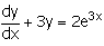is :

Detailed Solution for Test: Linear Differential Equations - Question 1

dy/dx + 3y = 2e3x
p = 3,    q = 2e3x
∫p.dx = 3x
Integrating factor (I.F) = e3x
y(I.F) = ∫Q(I.F) dx
ye3x = ∫2e3x e3x dx
ye3x = ∫2e6x dx
ye3x = 2∫e6x dx
ye3x = 2/6[e6x] + c
ye3x = ⅓[e6x] + c
Dividing by e3x, we get
y = ⅓[e3x] + ce-(3x)

Test: Linear Differential Equations - Question 2

### The integrating factor of differential equation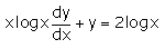is :

Detailed Solution for Test: Linear Differential Equations - Question 2

xlog x dy/dx + y = 2logx
⇒ dy/dx + y/(xlogx) = 2/x...(1)
Put P = 1/x logx
⇒∫PdP = ∫1/x logx dx
= log(logx)
∴ I.F.= e∫PdP = elog(logx)
= logx

Test: Linear Differential Equations - Question 3

### The solution of the differential equation x dy = (2y + 2x4 + x2) dx is:​

Detailed Solution for Test: Linear Differential Equations - Question 3

xdy = (2y + 2x4 + x2)dx
→ dy/dx − (2x)y = 2x3 + x
This differential is of the form y′+P(x)y=Q(x) which is the general first order linear differential equation, where P(x) and Q(x) are continuous function defined on an interval.
The general solution for this is y∙I.F = ∫I.F × Q(x)dx
Where I.F = e∫P(x)dx is the integrating factor of the differential equation.
I.F = e∫P(x)dx
= e∫−2/xdx
= e(−2∙lnx)
= eln(x−2)
= x−2
Thus y(1/x2) = ∫1/x2(2x3 + x)dx
=∫(2x + 1/x)dx
= x2 + lnx + C
⟹ y = x4 + x2lnx + c

Test: Linear Differential Equations - Question 4

The solution of the differential equation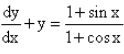Test: Linear Differential Equations - Question 5

The integrating factor of differential equation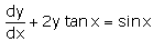is :

Detailed Solution for Test: Linear Differential Equations - Question 5

dy/dx+2ytanx=sinx
This is in the form of dy/dx + py = θ
where p=2tanx,θ=sinx
∴ finding If e∫pdx = e∫2tanxdx
=e(2log secx)
=e(log sec2x)
=sec2x

Test: Linear Differential Equations - Question 6

The solution of the differential equation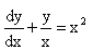is :

Detailed Solution for Test: Linear Differential Equations - Question 6

dy/dx + y/x = x2
differential equation is in the form : dy/dx + Py = Q
P = 1/x      Q = x2
I.F = e∫P(x)dx
I.F = e∫1/x dx
I.F = e[log x]
I.F = x
y I.F = ∫(Q * I.F) dx + c
yx = ∫x2 * x * dx + c
yx = ∫x3 dx + c
xy = [x4]/4 + c

Test: Linear Differential Equations - Question 7

The solution of the differential equation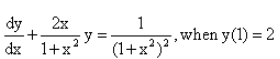is :

Test: Linear Differential Equations - Question 8

The solution of the differential equation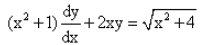is :

Detailed Solution for Test: Linear Differential Equations - Question 8

The given differential equation may be written as
dy/dx + (2x/(x2+1)y = √x2+4/(x2+1)  ... (i)
This is of the form dy/dx + Py = Q,
where P=2x/(x2+1) and Q=(√x2+4)/(x2+1)
Thus, the given differential equation is linear.
IF=e(∫Pdx)
= e(∫2x(x2+1)dx)
= e(log(x2+1) = (x2+1)
So, the required solution is given by
y × IF = ∫{Q×IF}dx + C,
i.e., y(x2+1)=∫(√x2+4)/(x2+1)×(x2+1)dx
⇒y(x2+1)=∫(√x2+4)dx
=1/2x (√x2+4) +1/2 × (2)2 × log|x+(√x2+4)| + C
=1/2x (√x2+4) + 2log|x+(√x2+4) + C.
Hence, y(x2+1) = 1/2x(√x2+4) + 2log|x+√x2+4| + C is the required solution.

Test: Linear Differential Equations - Question 9

The integrating factor of differential equation ​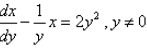is :

Test: Linear Differential Equations - Question 10

The integrating factor of differential equation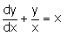is :​

Detailed Solution for Test: Linear Differential Equations - Question 10

dy/dx + y/x = x
Differential eq is in the form of : dy/dx + Py = Q
P = 1/x     Q = x
I.F. = e∫Pdx
= e∫(1/x)dx
= e(log x)
⇒ x

## Mathematics (Maths) Class 12

209 videos|209 docs|139 tests
 Use Code STAYHOME200 and get INR 200 additional OFF Use Coupon Code
Information about Test: Linear Differential Equations Page
In this test you can find the Exam questions for Test: Linear Differential Equations solved & explained in the simplest way possible. Besides giving Questions and answers for Test: Linear Differential Equations, EduRev gives you an ample number of Online tests for practice

## Mathematics (Maths) Class 12

209 videos|209 docs|139 tests